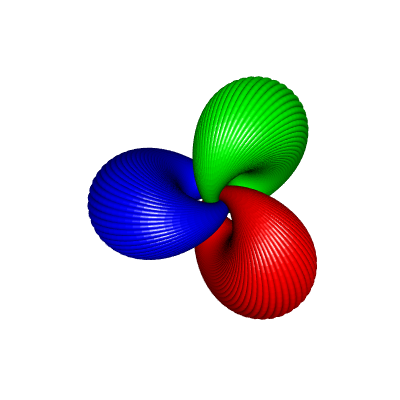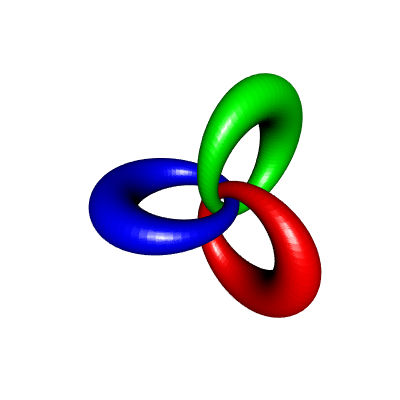Posted on June 3, 2018 by Stéphane Laurent

In this post, I will show how to construct some linked cyclides and draw them in R with rgl. The construction is based on the Hopf fibration. To draw a cyclide, the method is the following one: for a given $$\phi \in [-\pi/2, \pi/2)$$ and for every $$\theta \in [0, 2\pi)$$,

• take the Hopf fiber corresponding to the point on the two-dimensional sphere $$S^2$$ with spherical coordinates $$(\theta, \phi)$$, which is a great circle of the three-dimensional sphere $$S^3$$;

• rotate it in the four-dimensional space;

• apply the stereographic projection to the rotated fiber.

This gives a circle in the three-dimensional space, and the union of the circles over $$\theta \in [0, 2\pi)$$ forms a cyclide.

Now, if you repeat the construction with another rotation, the two cyclides you get are linked (see the pictures below).

To draw a circle with rgl, we will actually draw the torus whose equator is this circle, with a small minor radius. To do so, we will use the functions createTorusMesh and transfoMatrix that I introduced in a previous post. The code is also available in this gist.

Now, here is the promised code.

# load the functions createTorusMesh and transfoMatrix
source("TorusPassingByThreePoints.R")

# "inverse" Hopf map
hopfinverse <- function(q, t){
1/sqrt(2*(1+q)) * c(q*cos(t)+q*sin(t),
sin(t)*(1+q),
cos(t)*(1+q),
q*sin(t)-q*cos(t))
}
# stereographic projection
stereog <- function(x){
c(x, x, x)/(1-x)
}
# rotation in 4D space (right-isoclinic)
rotate4d <- function(alpha, beta, xi, vec){
a = cos(xi)
b = sin(alpha)*cos(beta)*sin(xi)
c = sin(alpha)*sin(beta)*sin(xi)
d = cos(alpha)*sin(xi)
p = vec; q = vec; r = vec; s = vec
c(a*p - b*q - c*r - d*s,
a*q + b*p + c*s - d*r,
a*r - b*s + c*p + d*q,
a*s + b*r - c*q + d*p)
}

nCirclesByCyclide <- 100
theta_ <- seq(0, 2*pi, len=nCirclesByCyclide+1)[-1]
nCyclides <- 3
beta0_ <- seq(0,2*pi,length.out=nCyclides+1)[-1]
colors <- rainbow(nCyclides)
phi <- 1 # -pi/2 < phi < pi/2; close to pi/2 <=> big hole

library(rgl)
open3d(windowRect=c(50,50,450,450))
view3d(90,0)
for(i in 1:nCyclides){
beta0 <- beta0_[i]
for(theta in theta_){
# take 3 points on the Hopf fiber of the point with
# spherical coordinates (theta,phi), and rotate them
circle4d3pts <- sapply(c(0,2,4), function(t){
rotate4d(pi/2, beta0, 1,
hopfinverse(c(cos(theta)*cos(phi),
sin(theta)*cos(phi),
sin(phi)), t))
})
# apply the stereographic projection
# this gives 3 points on a circle in the 3D space
circle3d3pts <- apply(circle4d3pts, 2, stereog)
# draw the torus passing by these three points
mr <- transfoMatrix(circle3d3pts[,1], circle3d3pts[,2], circle3d3pts[,3])
tmesh <- transform3d(createTorusMesh(R=mr$radius, r=0.2), mr$matrix)
}
}

This code generates the following picture:If you increase $$\phi$$, this gives cyclides with a biggest hole, with a shape closer to the one of an ordinary torus. For example, here is the result for $$\phi=1.4$$:I used the same technique to do these animations with POV-Ray: